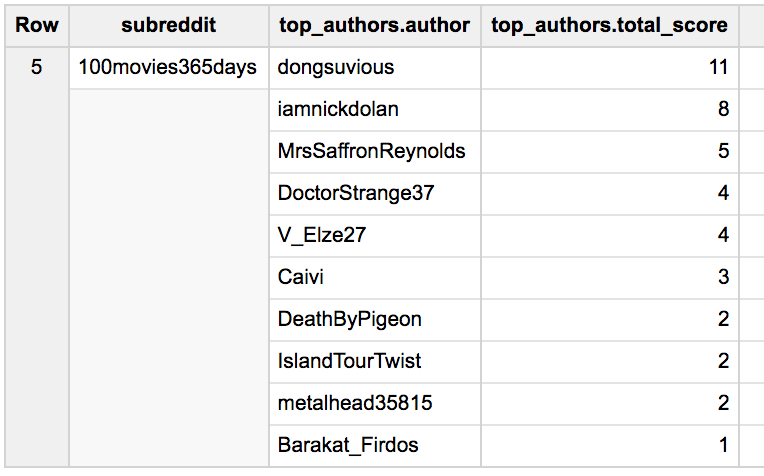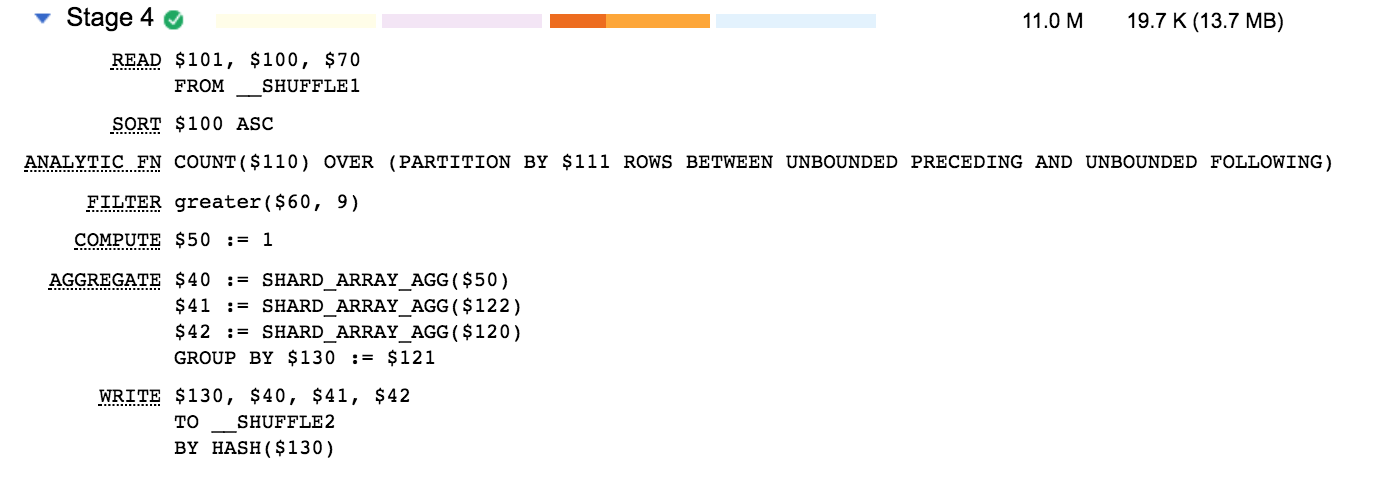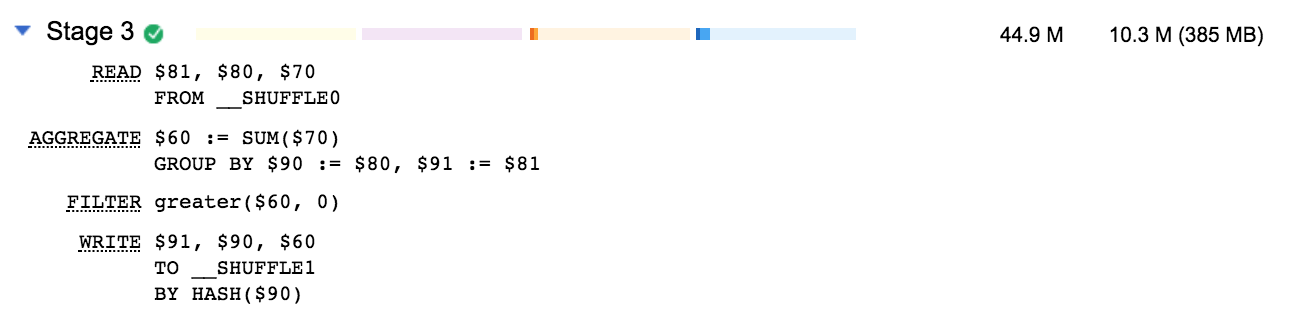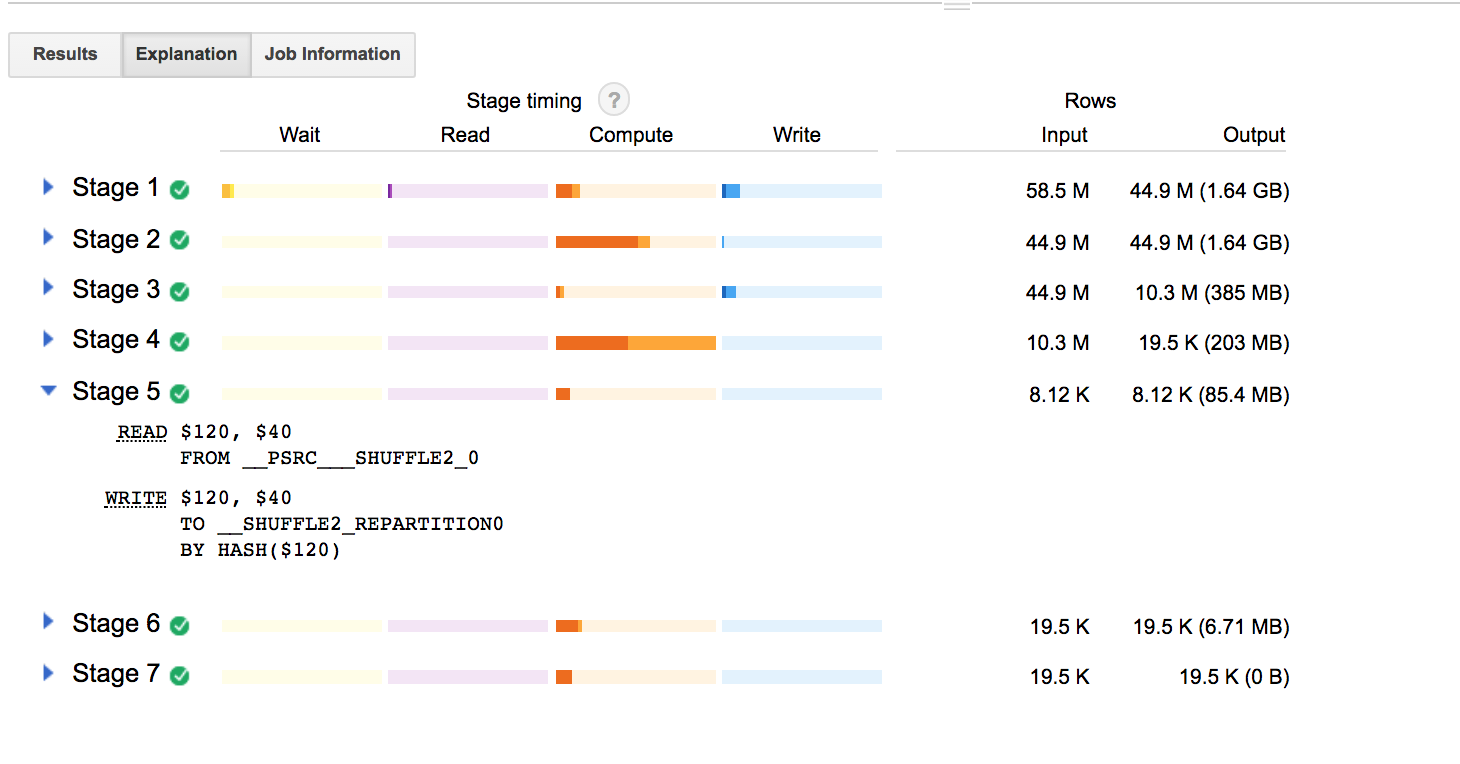# More Efficient Solutions to the Top N per Group Problem

In my last post, I tried to tackle getting the Top N Results per Group from a BigQuery dataset.

When I tweeted out the post, I got some great feedback and suggestions for more efficient ways to get the same results, so in this post I want to try to understand why the alternatives are more efficient.

## First Attempt using ROW_NUMBER

Here’s the initial query I was using:

``````SELECT
subreddit,
author,
author_count,
total_score,
comment_rank
FROM (
SELECT
subreddit,
author,
SUM(score) AS total_score,
ROW_NUMBER() OVER (PARTITION BY subreddit ORDER BY SUM(score) DESC) AS comment_rank,
COUNT(DISTINCT author) OVER (PARTITION BY subreddit) AS author_count
FROM
WHERE
author NOT IN ('[deleted]',
'AutoModerator')
GROUP BY
1,
2 )
WHERE
comment_rank <= 10
AND author_count > 9
ORDER BY
1,
5
``````

For the Top N problem, the key is computing the rank of each author’s scores, relative to the others in any particular group. In the query above, that’s this line:

``````ROW_NUMBER() OVER (PARTITION BY subreddit ORDER BY SUM(score) DESC) AS comment_rank,
``````

The partition isolates the group, in this case a single subreddit. Then, you can order the authors by score within each group. The `ROW_NUMBER` function is a way to capture an ordered list of those partitioned ranks.

We use the ordered list position later in the main select as part of the `WHERE` clause to only take author’s whose subreddit-specific comment rank is in the top 10.

Here’s what the query plan looks like for our `ROW_NUMBER` query:## USING ARRAY_AGG

The next query that I wanted to try was suggested by Elliot Brossard who actually works on BigQuery at Google. And, if I had been paying better attention when I pulled my first pass solution from StackOverflow, I would have seen that he also made this suggestion on the original post. Thanks for your patience, Elliot!

Elliot suggests using `ARRAY_AGG` with a limit to get a set of ranked results instead of using the `ROW_NUMBER` function:

``````SELECT
subreddit,
ARRAY_AGG(STRUCT(author,
total_score)
ORDER BY
total_score DESC
LIMIT
10) AS top_authors
FROM (
SELECT
subreddit,
author,
SUM(score) AS total_score,
COUNT(DISTINCT author) OVER (PARTITION BY subreddit) AS author_count
FROM
WHERE
author NOT IN ('[deleted]',
'AutoModerator')
GROUP BY
1,
2 )
WHERE
author_count > 9
GROUP BY
subreddit
ORDER BY
1
``````

ARRAY_AGG gives you a ton of power to condense parts of what we did in the first query.

• you can pull a subset of data into a single array, in this case `STRUCT(author, total_score)`, which gives you pretty much what we used `PARTITION` for in the first query
• `ARRAY_AGG` takes `ORDER BY` and `LIMIT` clauses, which together gives us the “top N” piece

Not only that, but the result set is reduced by an order of magnitude. The first query returns 196,970 results, or 10 rows per subreddit. But the `ARRAY_AGG` query only has 19,697 results because we only need one row per subreddit – the authors and there comment scores, per the `STRUCT` we defined in the `SELECT` statement are a single array value column.

Results using ROW_NUMBERResults using ARRAY_AGGAnd check out the query plan for the `ARRAY_AGG` query:Now, the first 3 stages look pretty similar in terms of row input/output sizes and distribution of wait/read/compute/write. Both plans are working on the sub-select at this point, doing the sum of author comments, grouped by subreddit.

Stage 4 gets really interesting. The `ARRAY_AGG` query takes an row size input of 11.0 M and reduces the output to 19.7K.In contrast, the `ROW_NUMBER` query does a bunch of compute on stage 4, but doesn’t reduce the output at all. It’s not until the `FILTER` in the next stage that we’ve cut down on the number of rows in the output (which we already know will still be 10x the `ARRAY_AGG` size).It looks like using `ROW_NUMBER` forces us to:

• run over all the data again to generate our “ordered list” of comment rankings
• rank everything before we filter out subsets of results we don’t ultimately want

In contrast to the other query strategies, this is starting to seem like wasted compute effort.

## USING APPROX_TOP_SUM

One more suggestion I got was to use the `APPROX_TOP_` functions. In this case `APPROX_TOP_SUM` seemed to do the trick:

``````SELECT
subreddit,
APPROX_TOP_SUM(author,
total_score,
10) AS top_scores
FROM (
SELECT
subreddit,
author,
SUM(score) AS total_score,
COUNT(DISTINCT author) OVER (PARTITION BY subreddit) AS author_count
FROM
WHERE
author NOT IN ('[deleted]',
'AutoModerator')
GROUP BY
1,
2
HAVING
SUM(score) > 0 )
WHERE
author_count > 9
GROUP BY
1
ORDER BY
1
``````

In the BigQuery documentation for this function it says that `APPROX_TOP_SUM` “returns the approximate top elements of `expression`, based on the sum of an assigned `weight`. The `number` parameter specifies the number of elements returned.

If the weight input is negative or NaN, this function returns an error.

Emphasis added to point out that, of course, since we’re dealing with reddit comments, some of them have been downvoted to hell. Negative comment scores will actually break the `APPROX_TOP_SUM` function in this case.

There are over 200 subreddits in this dataset where the top-ranked commenters couldn’t break into positive comment counts. I had to add a `HAVING` clause to only grab total scores above 0.

You can see that filter getting applied in stage 3:Compare that to the previous queries and you can see that stage 3 is where the total amount of data shrinks, thanks to those negative-ranked comments. But even with that caveat, it looks like `APPROX_TOP_SUM` performs similarly to `ARRAY_AGG`. In fact, I went back to the `ARRAY_AGG` query and adding the same filter on `SUM(score)` just to be sure that the inputs and outputs would match at each stage for both queries. They do.

Still, there are a few differences to point out for `APPROX_TOP_SUM`:

• why there is an additional stage (highlighted in the screenshot below) that seems to just be shuffling or repartitioning based on some kind of metadata?
• how does the ‘approximate’ part of `APPROX_TOP_SUM` factor into the computation?Other than that, it seems like both of these options deliver more concise results than the initial `ROW_NUMBER` solution. I’m looking forward to understanding the query explanation tools even better, so that in the future I can use them to diagnose my less efficient queries!